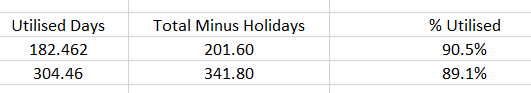# New to QlikView

Discussion board where members can get started with QlikView.

Contributor

## calculate percentages - am stuck!

Hello

maybe because its a friday but I am stuck trying to figure this out!

I have managed to build differing measures which are correct but when I try to calculate the percentage based on the measures its not providing the correct output! I have performed the logic in excel but cant get it right in the expressions i am building, i know its probably something simple but I cant figure it out. see below:i am expecting the following values though (excel screenshot below).My expressions are:

Utilised Days - =Sum( {\$<Usage = {"Utilised"}>} Days )

Total Days Minus Holidays - =Sum(Days)- Sum( {\$<Method = {"Holiday"}>} Days )

% Utilised = =Sum( {\$<Usage = {"Utilised"}>} Days )/Sum(Days)- Sum( {\$<Method = {"Holiday"}>} Days )/100

Any help much appreciated.

thanks Martin

1 Solution

Accepted SolutionsMVP

## Re: calculate percentages - am stuck!

Try this

=Sum( {\$<Usage = {"Utilised"}>} Days )/ (Sum(Days)- Sum( {\$<Method = {"Holiday"}>} Days ))/100

2 RepliesMVP

## Re: calculate percentages - am stuck!

Try this

=Sum( {\$<Usage = {"Utilised"}>} Days )/ (Sum(Days)- Sum( {\$<Method = {"Holiday"}>} Days ))/100

Contributor

## Re: calculate percentages - am stuck!

Thanks Sunny! I knew it was something simple!